NCERT Solutions for Class 7 Maths Chapter 15 Visualising Solid Shapes Ex 15.2 are part of NCERT Solutions for Class 7 Maths. Here we have given NCERT Solutions for Class 7 Maths Chapter 15 Visualising Solid Shapes Ex 15.2.

 Board CBSE Textbook NCERT Class Class 7 Subject Maths Chapter Chapter 15 Chapter Name Visualising Solid Shapes Exercise Ex 15.2 Number of Questions Solved 5 Category NCERT Solutions

## NCERT Solutions for Class 7 Maths Chapter 15 Visualising Solid Shapes Ex 15.2

Question 1.
Use isometric dot paper and make an isometric sketch for each one of the given shapes :Solution:Question 2.
The dimensions of a cuboid are 5 cm, 3cm and 2cm. Draw three different isometric sketches of this cuboid
Solution: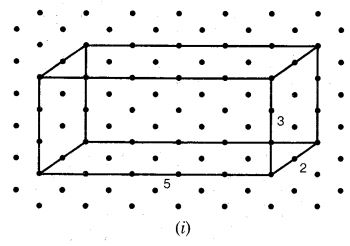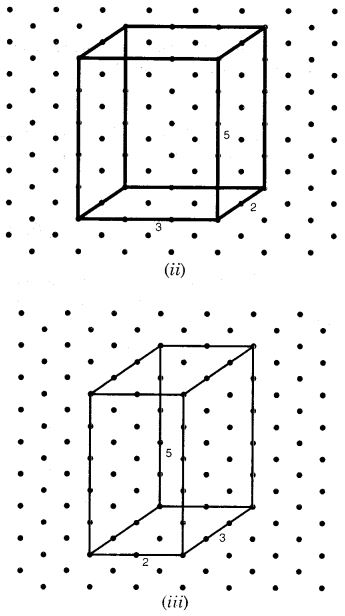Question 3.
Three cubes each with 2 cm edge are placed side by side to for,n a cuboid. Sketch an oblique or isometric sketch of this cuboid.
Solution: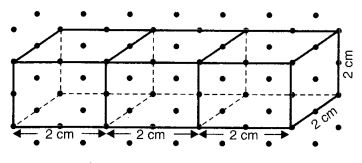Question 4.
Make an oblique sketch for each one of the given isometric shapes :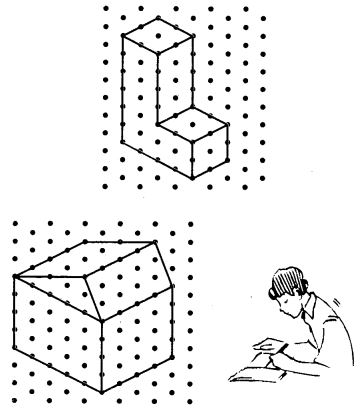Solution: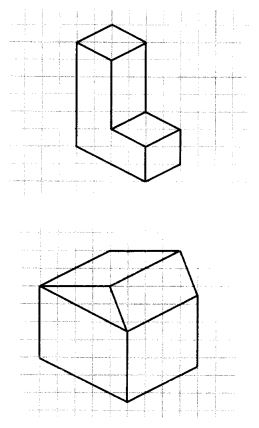Question 5.
Give (i) an oblique sketch and (ii) an isometric sketch for each of the following:
(a) A cuboid of dimensions 5 cm, 3 cm and 2 cm. (Is your sketch unique?)
(b) A cube with an edge 4 cm long.
Solution: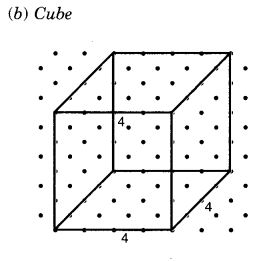We hope the NCERT Solutions for Class 7 Maths Chapter 15 Visualising Solid Shapes Ex 15.2 help you. If you have any query regarding NCERT Solutions for Class 7 Maths Chapter 15 Visualising Solid Shapes Ex 15.2, drop a comment below and we will get back to you at the earliest.